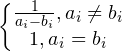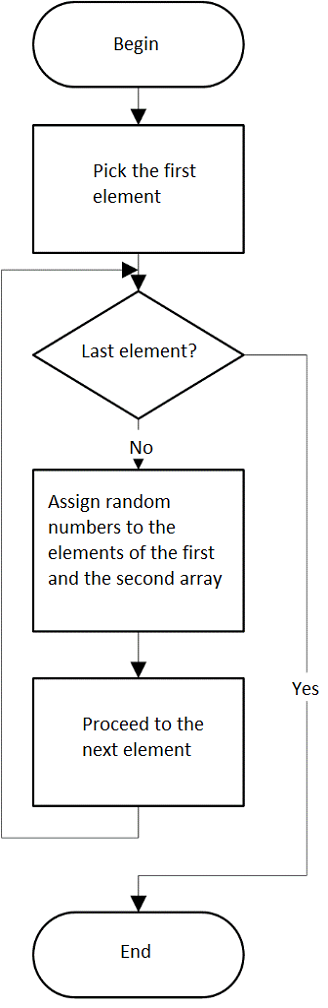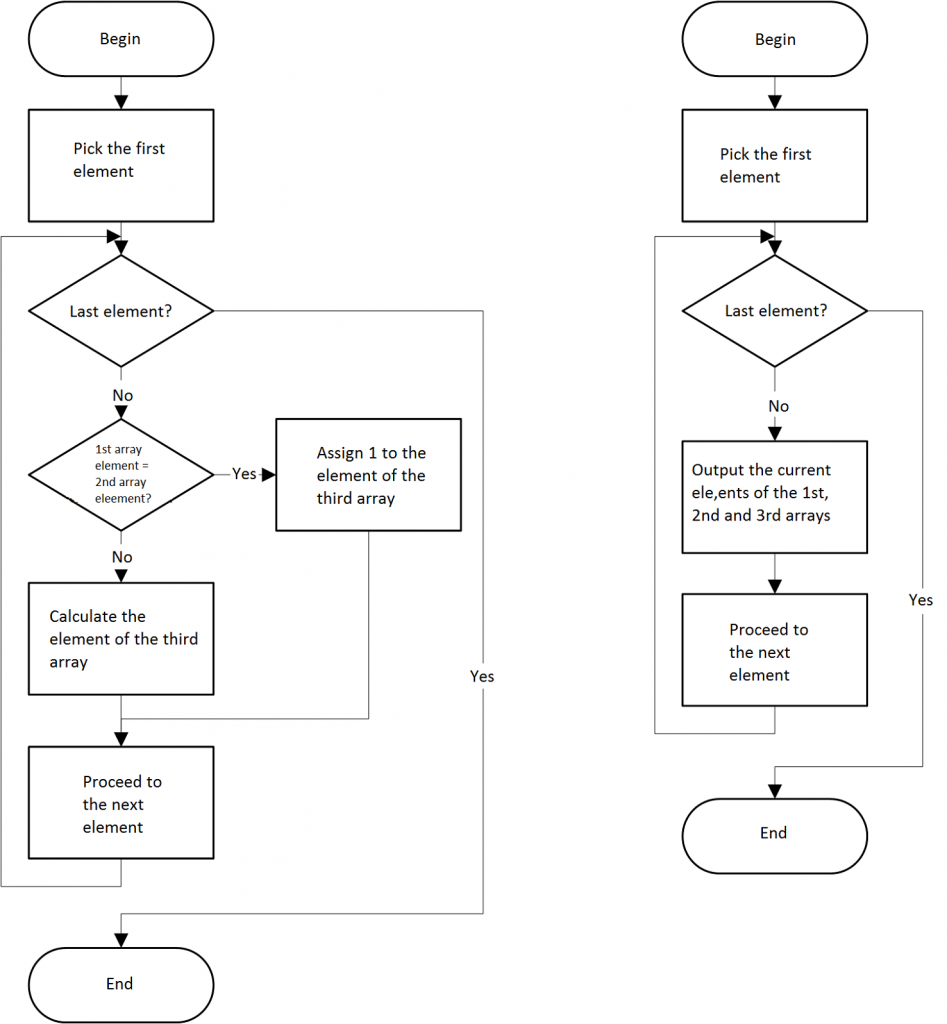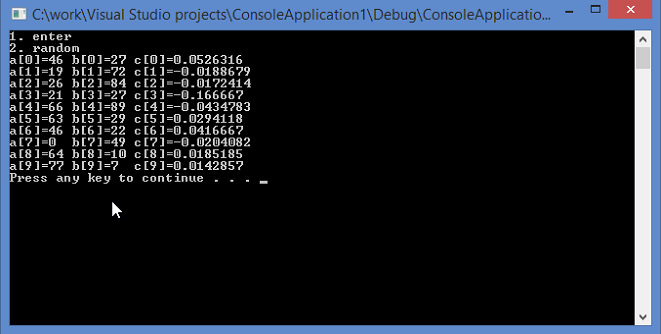# Sample Assignment: One-Dimentional Arrays

One-dimensional arrays A and B both contain 10 numbers. Build an array C, each element of which is calculated using the formula:Block-schemes of the solution:C++ implementation:

#include<iostream>
#include<time.h>
#include<conio.h>//for getch()

using namespace std;

int n = 10;//array size
bool flag = 1;

void random(int*a, int*b)//generate array of random numbers
{
for (int k = 0; k<n; k++)
{
a[k] = rand() % 100;//from 0 to 99
b[k] = rand() % 100;
}
}
void input(int*a, int*b)//input arrays manually
{
for (int k = 0; k<n; k++)
{
cout << "a[" << k << "]="; cin >> a[k];
cout << "b[" << k << "]="; cin >> b[k];
}
}
void calc(int*a, int*b, float*c)//calculate array c[]
{
for (int k = 0; k<n; k++)
c[k] = (a[k] == b[k]) ? 1 : 1 / ((float)a[k] - (float)b[k]);//if a==b, c=1; else c=1/(a-b)
}
void output(int*a, int*b, float*c)//output a[], b[], c[](result)
{
for (int k = 0; k<n; k++)
cout << "a[" << k << "]=" << a[k] << '\t' << "b[" << k << "]=" << b[k] << '\t' << "c[" << k << "]=" << c[k] << endl;
}
void main()
{
srand(time(0));//for random()
int *a = new int[n];//dynamic array
int *b = new int[n];//dynamic array
float *c = new float[n];//dynamic result array

int key;//switcher
do
{
cout << "1. enter\n2. random\n";
key = _getch();
switch (key)//switching
{
case '1': input(a, b); flag = 0; break;//manual input
case '2': random(a, b); flag = 0; break;//random input
default: cout << "you entered other number" << endl;
}
} while (flag);
calc(a, b, c); //calculate third massive
output(a, b, c); //output three massives
system("pause");//pause console
}


Screenshots:

Manual inputRandom inputThis sample assignment was completed by an expert from AssignmentShark. If you are interested in completing a task that concern one-dimensional arrays, apply to us for help. How to do this? Just fill the order form accurately, choose the best expert for you, and get exactly what you want. To choose the most appropriate expert you should examine the rating and experts’ profiles where you can find the information about knowledge and experience of experts in the specific fields. This sample assignment is not the single on our website. Take a look at more samples to make more comprehensive opinion about our service. Do not hesitate to make an order! Contact us as soon as possible!

Here is one more assignment example you may also find helpful – https://assignmentshark.com/blog/assignment-example-sample-on-how-to-start-programming-in-c/